# The GLMPOWER Procedure

### MANOVA Statement

Subsections:
• MANOVA ’label’ <test-options> </ detail-options>;

If the MODEL statement includes more than one dependent variable, you can indicate a multivariate model and define transformations of dependent variables by using the MANOVA statement.

The MANOVA statement enables you to define custom Type III hypothesis tests by specifying an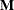vector or matrix for testing the hypothesis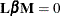. The
matrix consists of one or more between-subject contrasts that involve the model effects, and thematrix consists of one or more within-subject contrasts.

To use this feature, you must be familiar with the details of multivariate model and contrast parameterizations that are used in PROC GLM. For more information, see the sections Multivariate Analysis of Variance and Repeated Measures Analysis of Variance in Chapter 46: The GLM Procedure. For information about the power and sample size computational methods and formulas, see the section Contrasts in Fixed-Effect Multivariate Models.

You can use either the MANOVA statement or the REPEATED statement with any of the tests for multivariate models that are supported in the MTEST= option in the POWER statement. For handling repeated measures on the same experimental unit, you would usually use the REPEATED statement instead of the MANOVA statement. But you can use the MANOVA statement in repeated measures situations, in addition to situations where you have clusters or multiple outcome variables. The differences between the MANOVA and REPEATED statements are as follows:

• You can use the MANOVA statement to construct anymatrix, but you must specify the coefficients explicitly (except for the default identity matrix).

• You can use the REPEATED statement to specify commonly used contrasts by using keywords rather than coefficients, but you are limited to only those forms of thematrix.

There is no limit to the number of MANOVA statements that you can specify. Each power analysis includes tests for all MANOVA statements.

The label identifies the dependent variable transformation in the output. The label serves the same purpose as the factor-name in the REPEATED statement, enabling you to use the MANOVA statement for tests of within-subject effects and within-subject-by-between-subject interactions. A label is required for every transformation that is specified. Labels must be enclosed in single or double quotation marks.

#### Test Options

You can specify the following test-option in the MANOVA statement:

M=equation, …, equation | (row-of-matrix, …, row-of-matrix)

specifies a transformation matrix for the dependent variables that are listed in the MODEL statement.

The equations in the M= specification are of the form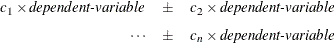where the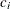values are coefficients of the various dependent-variables. If the value of a givenis 1, it can be omitted; in other words, 1Y is the same as Y. Equations should involve two or more dependent variables.

Alternatively, you can input the transformation matrix directly by entering the elements of the matrix, using commas to separate the rows and parentheses to surround the matrix. When you use this alternate form of input, the number of elements in each row must equal the number of dependent variables. Although these combinations actually represent the columns of thematrix, they are displayed by rows.

When you include an M= specification, the tests are based on the variables that are defined by the equations in the specification, not the original dependent variables. If you omit the M= option, the tests are based on the original dependent variables in the MODEL statement. Omitting the M= option is equivalent to specifying an identity matrix, as in the following example, which assumes three dependent variables:

MANOVA 'Identity' M=(1 0 0,
0 1 0,
0 0 1);


For more examples of the M= option, see the section Examples in Chapter 46: The GLM Procedure. The syntax and functionality of the M= option in PROC GLM are the same as in PROC GLMPOWER.

#### Detail Options

You can specify the following detail-option in the MANOVA statement after a slash (/):

ORTH

requests that the transformation matrix in the M= specification of the MANOVA statement be orthogonalized by rows before the analysis.